#### Satterthwaite Degrees of Freedom Approximation

The DDFM=SATTERTHWAITE option in the MODEL statement requests that denominator degrees of freedom in t tests and F tests be computed according to a general Satterthwaite approximation.

The general Satterthwaite approximation computed in PROC GLIMMIX for the test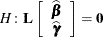is based on the F statistic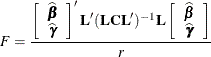where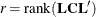and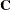is the approximate variance matrix of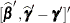. See the section Estimated Precision of Estimates and the section Aspects Common to Adaptive Quadrature and Laplace Approximation.

The approximation proceeds by first performing the spectral decomposition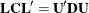, where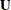is an orthogonal matrix of eigenvectors and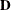is a diagonal matrix of eigenvalues, both of dimension. Define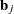to be the jth row of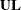, and let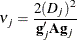where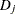is the jth diagonal element ofand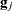is the gradient of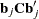with respect to, evaluated at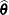. The matrix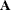is the asymptotic variance-covariance matrix of, which is obtained from the second derivative matrix of the likelihood equations. You can display this matrix with the ASYCOV option in the PROC GLIMMIX statement.

Finally, let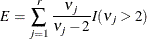where the indicator function eliminates terms for which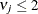. The degrees of freedom for F are then computed as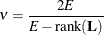provided E > r; otherwise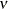is set to 0.

In the one-dimensional case, when PROC GLIMMIX computes a t test, the Satterthwaite degrees of freedom for the t statistic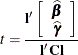are computed as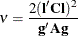where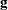is the gradient of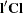with respect to, evaluated at.

The calculation of Satterthwaite degrees of freedom requires extra memory to hold q matrices that are the size of the mixed model equations, where q is the number of covariance parameters. Extra computing time is also required to process these matrices. The implemented Satterthwaite method is intended to produce an accurate F approximation; however, the results can differ from those produced by PROC GLM. Also, the small-sample properties of this approximation have not been extensively investigated for the various models available with PROC GLIMMIX.